Courses

# Sequences And Series Of Real Numbers -3

## 20 Questions MCQ Test Topic-wise Tests & Solved Examples for IIT JAM Mathematics | Sequences And Series Of Real Numbers -3

Description
This mock test of Sequences And Series Of Real Numbers -3 for Mathematics helps you for every Mathematics entrance exam. This contains 20 Multiple Choice Questions for Mathematics Sequences And Series Of Real Numbers -3 (mcq) to study with solutions a complete question bank. The solved questions answers in this Sequences And Series Of Real Numbers -3 quiz give you a good mix of easy questions and tough questions. Mathematics students definitely take this Sequences And Series Of Real Numbers -3 exercise for a better result in the exam. You can find other Sequences And Series Of Real Numbers -3 extra questions, long questions & short questions for Mathematics on EduRev as well by searching above.
QUESTION: 1

### If Sn denoted the sum of n term of the series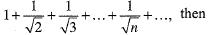Solution:

If Sn denote the sum of n terms of the series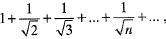then Sn will be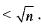QUESTION: 2

### Which of the following (s) is/are correct?

Solution: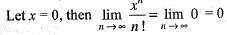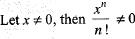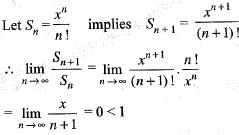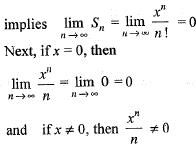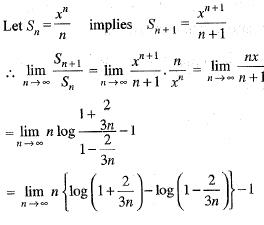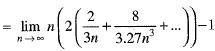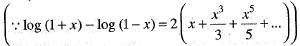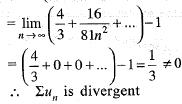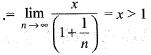(given)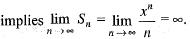QUESTION: 3

### Let < Sn > and <tn> be two sequences such that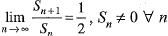and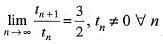Then,

Solution:

Since, we know that if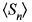be a sequence such that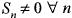and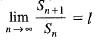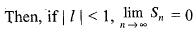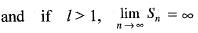Hence,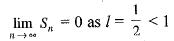and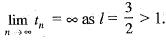*Multiple options can be correct
QUESTION: 4

The sequence <sn> , where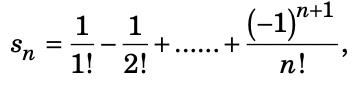is

Solution:

If m > n,Since 2r – 1 ≤ r !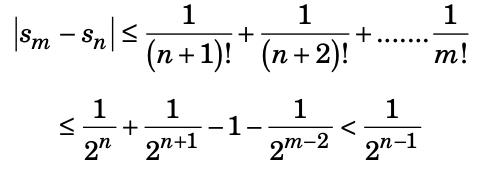Therefore, it follows that <sn> is a cauchy sequence. Hence <sn> converges

QUESTION: 5

Which one of the following is incorrect?

Solution:
QUESTION: 6

For infinite series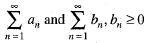for n, and there is a real number N, such that for n ≥ N implies |an|≤ bn.  If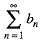coverges, then

Solution:
QUESTION: 7

If a sequence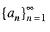converges to a real number A, then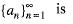Solution:
QUESTION: 8

If a sequence is not a Cauchy sequence, then it is a

Solution:
QUESTION: 9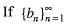is an increasing bounded sequence, then for the sequence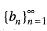is following statement is false

Solution:
QUESTION: 10

If a sequence < an2 > converges to a2, then the sequence < an > converges to

Solution:
QUESTION: 11

A real number l is a limit point of a sequence < an> if and only if there exists

Solution:
QUESTION: 12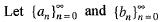be tw0 sequence such that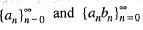converges respectively to A and AB, then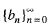converges Iff

Solution:
QUESTION: 13

Let sequence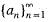converges to A and sequence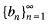converges to B, with an ≤ bn for all n, then

Solution:
QUESTION: 14

What will be the value of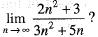Solution:
QUESTION: 15

A : Cauchy sequence is convergent.
B : Cauchy sequence is bounded.

Solution:
QUESTION: 16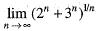is equal to

Solution:
QUESTION: 17

Let San be a convergent series of positive terms and let Sbn be a divergent series of positive terms. Then,

Solution:
QUESTION: 18

Which of the following statement is true? (a) For any positive number ε there is a natural number n, such that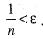. n (ft) Between any two real number there is no irrational number. (c) Convergent sequence is not bounded. (d) None of the above is true.

Solution:
QUESTION: 19

Let {an} be a sequence of real numbers. Then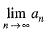exists if and only if

Solution:

For C, suppose lima2n = p, lim a2n+1 = q, lim a3n = r.

Considering a6n, p = r.

Considering a6n+3 = a2(3n+1)+1, q = r.

Therefore p=q=r.

Since a2n and a2n+1 include all integers, limanliman exists.

So C is true.

QUESTION: 20

Let < an> —> a. Let for every positive integer k, Ak be the set of all positive integer n such that | an- a | < —1/k. Then,

Solution: